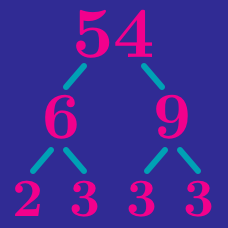Number Theory

# Prime Factorization and Divisors: Level 3 Challenges

Find the number of odd (positive) divisors of $7!$.

Find smallest positive $a$ for $a={ b }^{ 2 }={ c }^{ 3 }={ d }^{ 5 }$ such that $a,b,c,d$ are distinct integers.

$6128704063125000=2^3\times 3^5 \times 5^7 \times 7^9$

For the number above, find the number of divisors which are perfect squares.

For two non-negative integers $a$ and $b$ , the equation ${ 3\cdot 2 }^{ a }+1={ b }^{ 2 }$ has solutions $({ a }_{ 1 },{ b }_{ 1 }),({ a }_{ 2 },{ b }_{ 2 }),...,({ a }_{ n },{ b }_{ n })$.

Find ${ a }_{ 1 }{ +b }_{ 1 }+{ a }_{ 2 }+{ b }_{ 2 }+...+{ a }_{ n }+{ b }_{ n }$?

Given that $x^{2} + 5x + 6$ is a prime number, determine the smallest integer value of $x$.

Details and Assumptions:

• If you think no such solution exists, input $999$ as your answer.
×

Problem Loading...

Note Loading...

Set Loading...